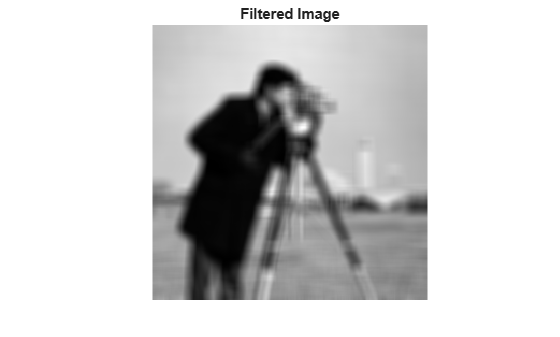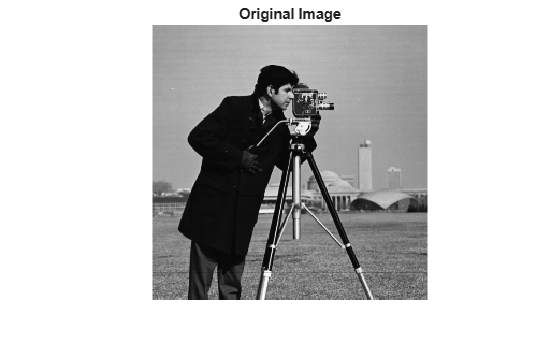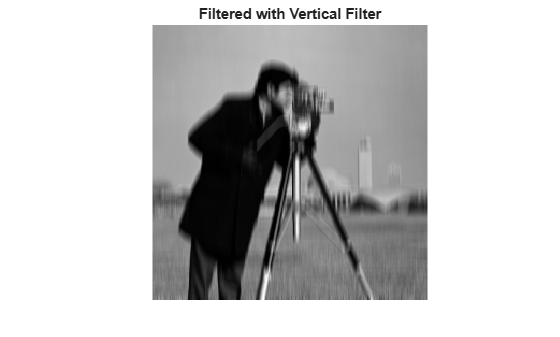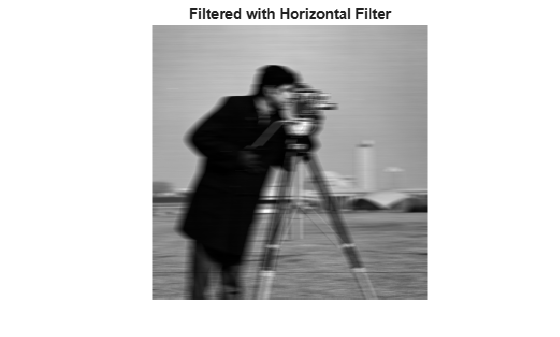# integralBoxFilter

2-D box filtering of integral images

## Syntax

``B = integralBoxFilter(A)``
``B = integralBoxFilter(A,filterSize)``
``B = integralBoxFilter(___,Name,Value)``

## Description

````B = integralBoxFilter(A)` filters the integral image `A` with a 3-by-3 box filter. Returns the filtered image, `B`. ```

example

````B = integralBoxFilter(A,filterSize)` filters the integral image `A` with a 2-D box filter with size specified by `filterSize`.```
````B = integralBoxFilter(___,Name,Value)` uses name-value pairs to control various aspects of the filtering.```

## Examples

collapse all

`A = imread('cameraman.tif');`

Pad the image by the radius of the filter neighborhood. This example uses an 11-by-11 filter.

```filterSize = [11 11]; padSize = (filterSize-1)/2; Apad = padarray(A, padSize, 'replicate','both');```

Compute the integral image of the padded input image.

`intA = integralImage(Apad);`

Filter the integral image.

`B = integralBoxFilter(intA, filterSize);`

Display original image and filtered image.

```figure imshow(A) title('Original Image')``````figure imshow(B,[]) title('Filtered Image')```` A = imread('cameraman.tif');`

Pad the image by radius of the filter neighborhood, calculated `(11-1)/2`.

```padSize = [5 5]; Apad = padarray(A, padSize, 'replicate', 'both');```

Calculate the integral image of the padded input.

`intA = integralImage(Apad);`

Filter the integral image with a vertical [11 1] filter.

`Bvert = integralBoxFilter(intA, [11 1]);`

Crop the output to retain input image size and display it.

`Bvert = Bvert(:,6:end-5);`

Filter the integral image with a horizontal [1 11] filter.

`Bhorz = integralBoxFilter(intA, [1 11]);`

Crop the output to retain input image size.

`Bhorz = Bhorz(6:end-5,:);`

Display the original image and the filtered images.

```figure, imshow(A) title('Original Image')``````figure, imshow(Bvert,[]) title('Filtered with Vertical Filter')``````figure, imshow(Bhorz,[]) title('Filtered with Horizontal Filter')```## Input Arguments

collapse all

Integral image to be filtered, specified as a numeric array of any dimension.

The integral image must be upright — `integralBoxFilter` does not support rotated integral images. The first row and column of the integral image is assumed to be zero-padded, as returned by `integralImage`.

Data Types: `double`

Size of box filter, specified as a positive, odd integer or 2-element vector of positive, odd integers. If `filterSize` is scalar, then `integralBoxFilter` uses a square box filter.

Data Types: `single` | `double` | `int8` | `int16` | `int32` | `int64` | `uint8` | `uint16` | `uint32` | `uint64`

### Name-Value Arguments

Specify optional pairs of arguments as `Name1=Value1,...,NameN=ValueN`, where `Name` is the argument name and `Value` is the corresponding value. Name-value arguments must appear after other arguments, but the order of the pairs does not matter.

Before R2021a, use commas to separate each name and value, and enclose `Name` in quotes.

Example: `B = integralBoxFilter(A,5,'NormalizationFactor',1);`

Normalization factor applied to box filter, specified as a numeric scalar.

The default `'NormalizationFactor'` has the effect of a mean filter — the pixels in the output image are the local means of the image. To get local area sums, set `'NormalizationFactor'` to `1`. To avoid overflow in such circumstances, consider using double precision images by converting the input image to class `double`.

Data Types: `single` | `double` | `int8` | `int16` | `int32` | `int64` | `uint8` | `uint16` | `uint32` | `uint64`

## Output Arguments

collapse all

Filtered image, returned as a numeric array. `integralBoxFilter` returns only the parts of the filtering that are computed without padding.

Data Types: `double`

## Version History

Introduced in R2015b